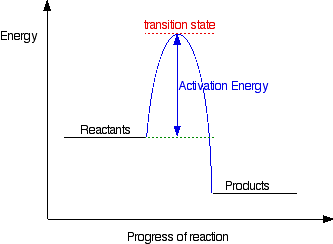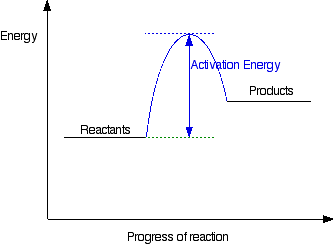# Chemical and Energy Changes

?

## Acids and AlkalisWhether a substance is an acid/alkali depends on type of ions released when substance dissolved in water. Acids from hydrogen ions (H+) when dissolved in water and alkalis form hydroxide ions (OH-)

1 of 12

## Neutralisation reactions

General equation for neutralisation reaction = acid + alkali --> salt + water

Neutralisation reactions between acids and alkalis can be shown in terms of H+ and OH- ions. H+ ions from acid react with OH- ions from alkali to produce water:

H+ +  OH-  -->  H2O

2 of 12

## Strong and Weak Acids

Acid Strength - tells you proportion of acid particles that'll dissociate to produce H+ ions in a solution.

Strong Acids - an acid (such as sulfuric, hydrochloric and nitrate acid) that completely dissociates its H+ ions in water (not a reversible reaction)

e.g. HCl(g) + H2O(l)→H+(aq) + Cl-(aq)

Weak Acidsan acid that only partially dissosiates its H+ ions in water (equilibrium-reversible reaction)

e.g. CH3COOH(aq)H+(aq) + CH3COO-(aq)

For every decrease of 1 on the pH scale the concentration of H+ ions increases by factor of 10. (x = difference in pH)

Factor H+ ion concentration changes by = 10-x

3 of 12

## Reactions of Acids

A base is a substance that can accept a hydrogen ion (H+) from another substance. They're usally metal oxides or metal hydroxides.

Acid + Metal oxide/hydroxide --> salt + water

Metal carbonates are also bases - react with acids to produce carbon dioxide

Metal Carbonate + acid --> metal salt + carbon dioxide + water

Acid + Metal -->  salt + hydrogen

4 of 12

## Reactivity of MetalsCarbon and Hydrogen (non-metals) included in reactivity series as they're used to displace metals from their ores.

*The reactivity of metals is derived from how easily it loses an electron and becomes a postive ion

5 of 12

## Reactivity of Metals...

Reactions that give evidence of reactivity = Metal + acid --> salt + hydrogen & Metal + water --> metal hydroxide + hydrogen

Displacement Reaction= Metal A + Metal B compound --> Metal B + Metal A compound (if A > B)

A more reactive metal will dispalce a less reactive metal from its compound. e.g. 2HCl + Mg --> MgCl2 + H2

6 of 12

## Acids and Carbonates

*Most chlorides, sulphates + nitrates are soluble (can be dissolved)

*Most oxides, hydroxides + carbonates are insoluble (can't be dissolved)

Ca(OH)2(aq) + CO2(g) --> CaCO3(s) + H2O(l)  ------ Precipitation reaction

*Precipitation reaction = when an insoluble solid is formed from a reaction

Precipitation reaction = (aq) + (aq) --> (s)  or  (g) + (aq) --> (s)

7 of 12

## Redox Reactions

Oxidation can be defined as the gain of oxygen by an element or compound  (1)

e.g. 2Mg + O2 --> 2MgO (*Mg has been oxidised - gained oxygen)

Reduction can be defined as the loss of oxygen by an element or compound (1)

e.g. 2CuO + C --> 2Cu + CO2 (*CuO has been reduced - lost oxygen)

Oxidation is the loss of electrons (2)

e.g. Zn(s) → Zn2+(aq) + 2e-

Reduction is the gain of electrons (2)

e.g. Cu2+(aq) + 2e-→ Cu(s)

Both oxidation and reduction happen at the same time - redox reaction - Cu2+(aq) + Zn(s)→Cu(s) + Zn2+(aq)

8 of 12

## Electrolysis

'Electro' = electricity (molten)

'Lysis' = split (solution - dissolved)

IONIC - if solid (lattice) *no free ions to pass current (so has to be molten)

Electrolyte: a liquid/gel that contain ions and can be decomposed by electrolysis

Anode (+) --> attracts negative ions - Anions go to Anode

Cathode (-) --> attracts positive ions - Cations go to Cathode

Rules for predicting products at Electrolysis in solutions

•  At negative electrode LEAST reactive ion loses electrons and forms an element
• At positive electrode if halides (group 7) present, halides will form
• At positive electrode if no halides present, OH- ions will produce O2
• At positive electrode if no halides or OH- ions present other negative ion will form its element
9 of 12

## Energy transfer in reactions

Exothermic

• surroundings get 'hotter'
• combustion (CH4 + 2O2 --> CO2 + 2H2O)
• hand warmers
• neutralisation

Exothermic energy profile10 of 12

## Energy transfer in reactions...

Endothermic

• suuroundings get 'colder' (loss of heat energy)
• ice packs11 of 12

## Energy transfer in reactions......

Activation Energy (Ea) = all reactions need a certain amount of energy to get started. This is called  Activation Energy.

Endothermic breaks bonds so energy is absorbed

Exothermic makes bonds so energy is released

Heat: energy in a system (measured in Joules)

Temp: Average profile motion in a system (measured in Farenheit, Celsius, Kelvin - impossible to reache 0 in Kelvin as all particles have at least some kinetic energy.

12 of 12